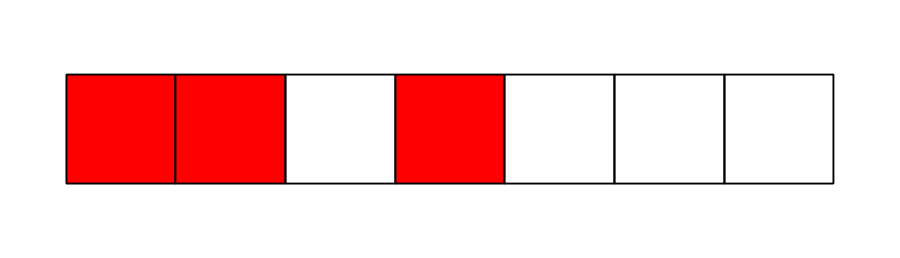# Flipped Colour

Alice wants Chris--a mischievous little boy--to deliver a message to Bob which consists of 7 squares, numbered 1 through 7 from left to right and colored either red or white. As expected, Chris switches the color of exactly one of the 7 squares in the original message and thus Bob receives the following message:Alice knew beforehand that Chris would play this trick, so she had already told Bob the following information on the original message:

• Squares numbered $1,3,5,7$ have an odd number of red squares.
• Squares numbered $2,3,6,7$ have an even number of red squares.
• Squares numbered $4,5,6,7$ have an odd number of red squares.

Which is the original message Alice wanted to send?

Details and assumptions:

• The options are expressed as binary numbers: $1$ represents a red square and $0$ represents a white square.
×